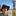# Paea Lagoon Aua i'a Fish Pond Monitoring, Group 3: Benthic Cover, Tahiti, FP, 2022

METHODS:
Substrate cover of the fish pond was measured using a 1 square meter quadrat for 20 preferentially sampled areas within the fish pond and 1 meter surrounding the outside perimeter of the fish pond walls. 20 total quadrats were taken within this sampling universe. Quadrat placement was measured on a grid of the fish pond, where we measured the quadrat distance from the southern most corner of the rock fish pond closest to the house wall (AKA the bottom left corner of the fish pond). However, for calculating quadrat distance considering the 1 meter extension of our sampling universe beyond the rock wall, we measured 1 meter south from the southern corner part to match the end of our sampling universe. We considered this spot the "origin" or starting point of all measurements at 0 meters in width and height. Quadrat placement was measured on the grid for replicability of future groups including 20 preferentially chosen placements. Substrate cover categories included coral, sand, coral rock, river rock, turf algae, and macro algae. The mean and standard deviation was calculated for each substrate category.

The quadrat locations are as follows including formatting of (Height, Width) in meters from the starting point of (0,0). These measurements are to the bottom left corner of the quadrat. Coordinates of our quadrats were: (5, 4.6), (7.5, 8.7), (13.3, 6.1), (10.8, 15.2), (14.1, 15.4), (9.2, 12.1), (2.8, 2.5), (5.5, 11.4), (8.1, 17.9), (15.6, 14.1), (4.4, 6.1), (7.2, 5.3), (9.7, 6.9), (14.4, 6.2), (13.8, 1), (7.1, 1.1), (12.5, 19), (11.3, 15.2), (5.6, 8.9), (3.1, 12.4)

For each quadrat two surveyors estimated the percent coverage for each quadrat peramater. The parameters evaluated were coral, sand, coral rock,river rock, turf algae, and macro algae cover. After field data collection, we calcuated the average percentages of each substrate between the two individual observations of the same quadrat to eliminate bias. Using these 20 average points for each type of substrate (coral, sand, coral rock, river rock, turf algae, and macro algae) we calculated the mean and standard deviation (S.D.) for each substrate as shared below.

DATA:
Coral: Mean = 10.125% and S.D. = 0.26
Sand: Mean = 49.425% and S.D. = 0.27
Coral Rock: Mean = 1.25% and S.D. = .028
River Rock: Mean = 16.35% and S.D. = 0.16
Turf Algae: Mean = 27.25% and S.D.= 0.20
Macro Algae: Mean = 1.8% and S.D. = 0.14

Based on these percentages the greatest to least percentages of substrate cover within the pond and 1 meter on the outside perimeter of the pond was sand, turf algae, river rock, coral, macro algae, and coral rock.

Publicado porlangzi, 06 de agosto de 2022, 01:09 AM

## Comentários

Nenhum comentário ainda.